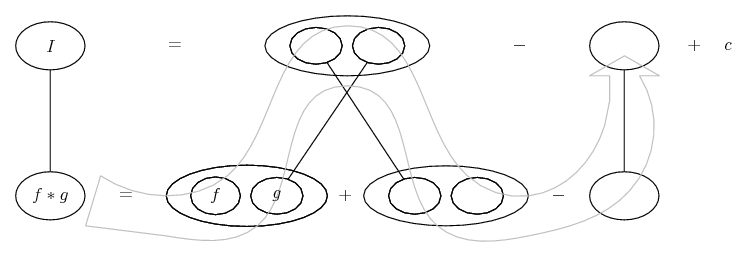# Thread: Need help with an integration problem

1. ## Need help with an integration problem

Here's what I'm working with:

$\displaystyle \int e^xcos(x)dx$

This does not appear to be a trivial problem, and I'm having trouble figuring out how to approach this. If I try integrating it by parts, I just end up with another integral that's very similar. Tossing this into WolframAlpha shows a *really* complicated u-sub. I've run into situations where WolframAlpha makes things a lot more complicated than they need to be. I'm hoping this is one of those times.Can anyone give me a hand?

2. Define it with its corresponding integral and integrate both by parts:

\displaystyle \begin{aligned} & I : = \int e^x\cos{x}\;{dx}, ~~ J := \int e^x \sin{x}\;{dx} \\& I = \int e^x \left(\sin{x}\right)'\;{dx} = e^x\sin{x}-\int e^x\sin{x}\;{dx} = e^x\sin{x}-J. \\& J = -\int e^x \left(\cos{x}\right)'\;{dx} = -e^x\cos{x}+\int e^x\cos{x}\;{dx} = -e^x\cos{x}+I. \end{aligned}

Now by abstracting these two integrals from each other, we have:

\displaystyle \begin{aligned} & I-J = e^x\sin{x}-J+e^x\cos{x}-I \\& \Rightarrow 2I = e^x\sin{x}+e^x\cos{x} \\& \Rightarrow I = \frac{1}{2}e^x \left(\sin{x}+\cos{x}\right). \end{aligned}

3. Definitely not a technique I'm familiar with. I'm going to have to stare at this for a while in order to comprehend it fully. Thank you for the insight!

4.Originally Posted by LancetHere's what I'm working with:

$\displaystyle \int e^xcos(x)dx$

This does not appear to be a trivial problem, and I'm having trouble figuring out how to approach this. If I try integrating it by parts, I just end up with another integral that's very similar. Tossing this into WolframAlpha shows a *really* complicated u-sub. I've run into situations where WolframAlpha makes things a lot more complicated than they need to be. I'm hoping this is one of those times.Can anyone give me a hand?
A 'suggestive' solution is based on the 'identity'...

$\displaystyle e^{x}\ \cos x = \frac{e^{(1+i) x} + e^{(1-i) x}}{2}$ (1)

... and You can integrate both terms of (1) using the standard rule...

$\displaystyle \int e^{a x} dx = \frac{e^{a x}}{a} + c$ (2)

Kind regards

$\displaystyle \chi$ $\displaystyle \sigma$

5.Originally Posted by LancetDefinitely not a technique I'm familiar with. I'm going to have to stare at this for a while in order to comprehend it fully. Thank you for the insight!
Just in case a picture helps (helps me by mapping out the relationships implied by combinations of relative pronouns, integral of, derivative of) ...

If you can buy this...Key:
Spoiler:... is the product rule: straight continuous lines differentiate downwards with respect to x.

... as lazy integration by parts, doing without u and v and du and dv (as does THC, too, to an extent) ... then you want 'integration by parts twice' i.e. filling the blanks here...... and then solving the top row for I.

Larger

__________________________________________________ __________

Don't integrate - balloontegrate!

Balloon Calculus; standard integrals, derivatives and methods

Balloon Calculus Drawing with LaTeX and Asymptote!

6. I believe I understand it now. Thanks for helping, everyone!

#### Search Tags

integration, problem